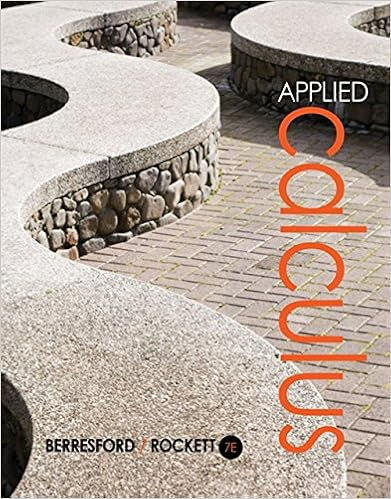# In the previous exercise we found that d dy gdy sech

• Homework Help
• 246
• 100% (3) 3 out of 3 people found this document helpful

This preview shows page 85 - 87 out of 246 pages.

##### We have textbook solutions for you!
The document you are viewing contains questions related to this textbook.The document you are viewing contains questions related to this textbook.
Chapter 6 / Exercise 72
Applied Calculus
Berresford/RockettExpert Verified
In the previous exercise we found that d dy gd(y) = sech y ; therefore, gd (y) = f (y) . Now, since the two functions have equal derivatives, they differ by a constant; that is, gd(y) = f (y) + C. To find C we substitute y = 0: tan 1 ( sinh 0 ) = 2tan 1 (e 0 ) π 2 + C tan 1 0 = 2tan 1 ( 1 ) π 2 + C 0 = 2 · π 4 π 2 + C C = 0 . Therefore, gd(y) = f (y). 47. Let t(y) = sinh 1 ( tan y) Show that t(y) is the inverse of gd(y) for 0 y < π/ 2. solution Let x = gd(y) = tan 1 ( sinh y) . Solving for y yields y = sinh 1 ( tan x) . Therefore, gd 1 (y) = sinh 1 ( tan y). 48. Verify that t(y) in Exercise 47 satisfies t (y) = sec y , and find a value of a such that t(y) = y a dt cos t solution Let t(y) = sinh 1 ( tan y) . Then t (y) = 1 cos 2 y tan 2 y + 1 = 1 cos 2 y 1 cos 2 y = 1 cos 2 y · 1 | cos y | = 1 | cos y | = | sec y | . For 0 y < π 2 , sec y > 0; therefore t (y) = sec y . Integrating this last relation yields t(y) t(a) = y a 1 cos t dt. For this to be of the desired form, we must have t(a) = sinh 1 ( tan a) = 0. The only value for a that satisfies this equation is a = 0.
##### We have textbook solutions for you!
The document you are viewing contains questions related to this textbook.The document you are viewing contains questions related to this textbook.
Chapter 6 / Exercise 72
Applied Calculus
Berresford/RockettExpert Verified
944 C H A P T E R 7 TECHNIQUES OF INTEGRATION 49.The relations cosh(it)=costand sinh(it)=isintwere discussed in the Excursion. Use these relations to show that the identity cos 2 t + sin 2 t = 1 results from setting x = it in the identity cosh 2 x sinh 2 x = 1. solution Let x = it . Then cosh 2 x = ( cosh (it)) 2 = cos 2 t and sinh 2 x = ( sinh (it)) 2 = i 2 sin 2 t = − sin 2 t. Thus, 1 = cosh 2 (it) sinh 2 (it) = cos 2 t ( sin 2 t) = cos 2 t + sin 2 t, as desired. 7.5 The Method of Partial Fractions Preliminary Questions 1. Suppose that f (x) dx = ln x + x + 1 + C . Can f be a rational function? Explain. solution No, f (x) cannot be a rational function because the integral of a rational function cannot contain a term with a non-integer exponent such as x + 1. 2. Which of the following are proper rational functions? (a)xx3(b)49x(c)x2+12(x+2)(x+1)(x3)(d)4x37x(x3)(2x+5)(9x) solution (a) No, this is not a proper rational function because the degree of the numerator is not less than the degree of the denominator. (b) Yes, this is a proper rational function. (c) Yes, this is a proper rational function. (d) No, this is not a proper rational function because the degree of the numerator is not less than the degree of the denominator. 3.Which of the following quadratic polynomials are irreducible? To check, complete the square if necessary. solution (a) Square is already completed; irreducible. (b) Square is already completed; factors as (x 5 )(x + 5 ) . (c) x 2 + 4 x + 6 = (x + 2 ) 2 + 2; irreducible. (d) x 2 + 4 x + 2 = (x + 2 ) 2 2; factors as (x + 2 2 )(x + 2 + 2 ) . 4. Let P/Q be a proper rational function where Q(x) factors as a product of distinct linear factors (x a i ) . Then P (x) dx Q(x) (choose the correct answer): (a) is a sum of logarithmic terms A i ln (x a i ) for some constants A i .
•••